用户名 Email 自动登录 找回密码 密码 立即注册

1.关注和取消关注用户

2. 判断与用户之间的关注关系

## 实现效果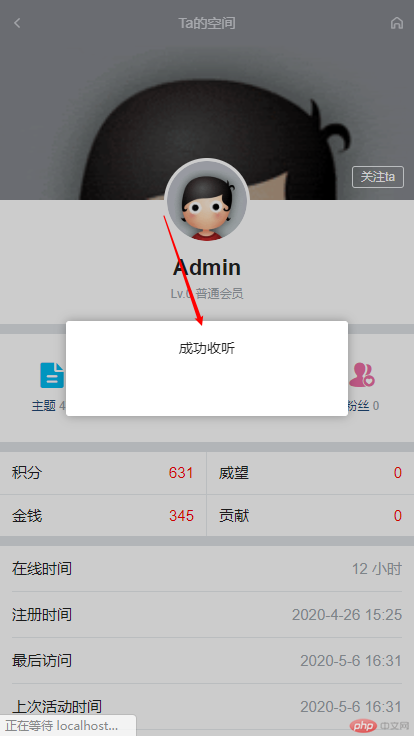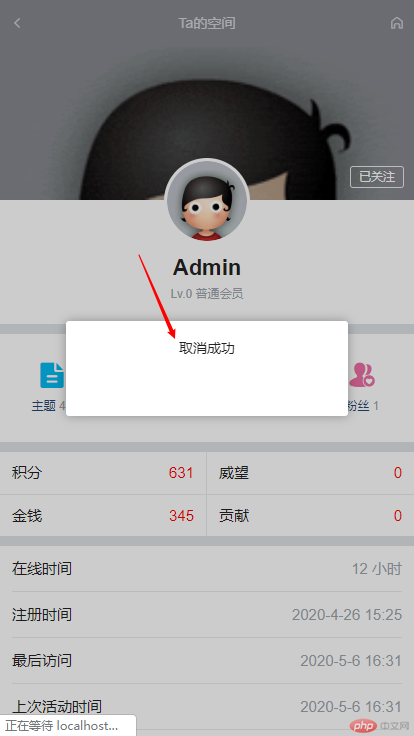## 实现方案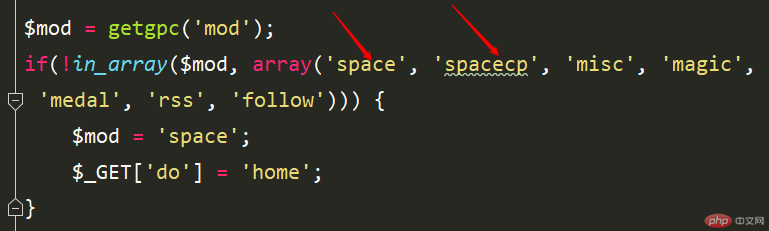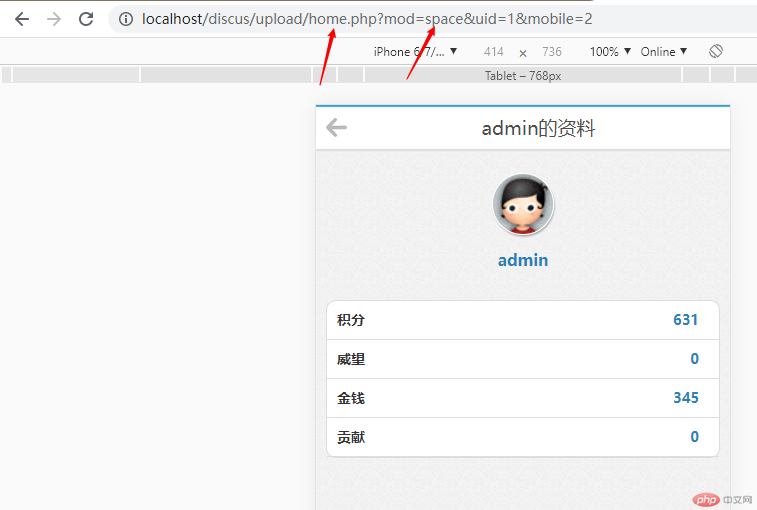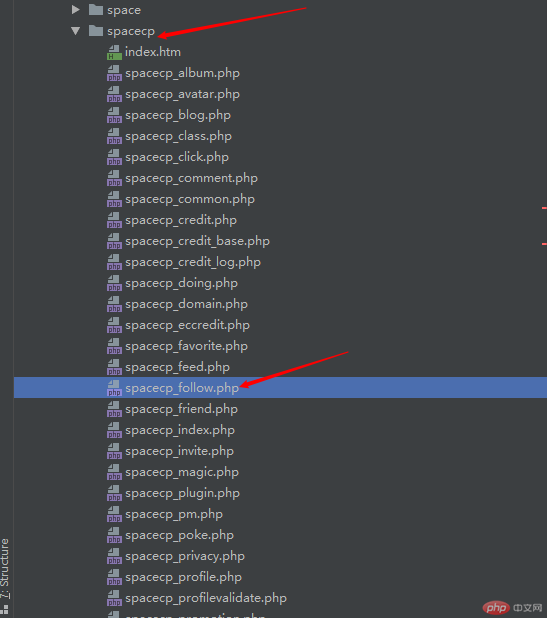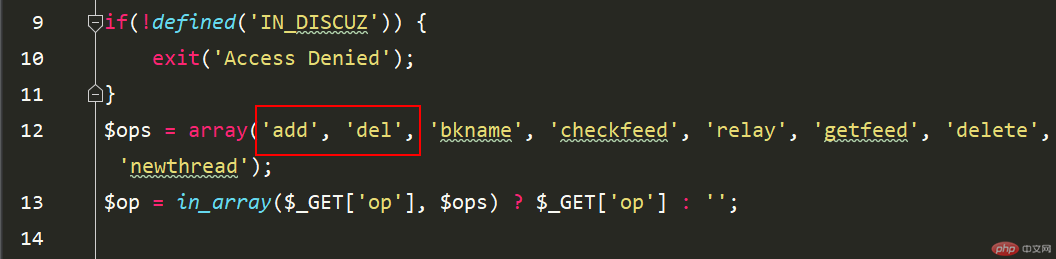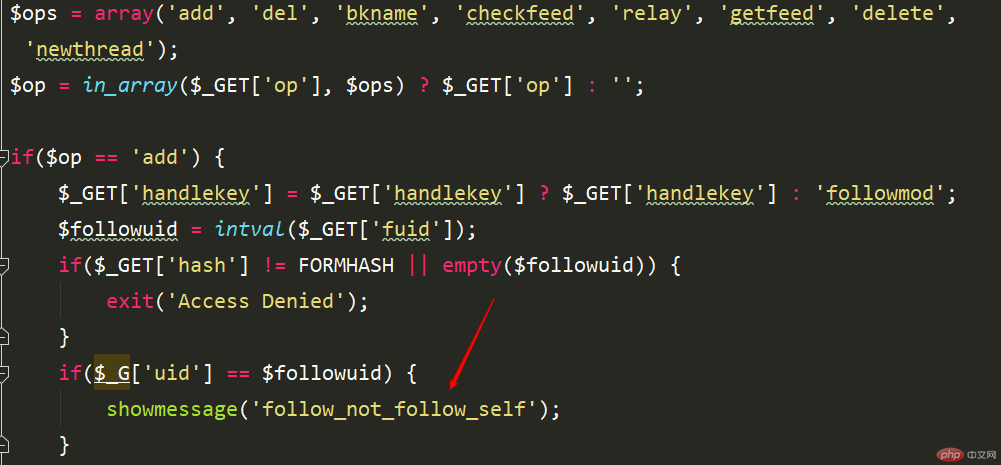`home.php?mod=spacecp&ac=follow&op=add&hash={FORMHASH}&fuid={\$space[uid]}&mobile=2`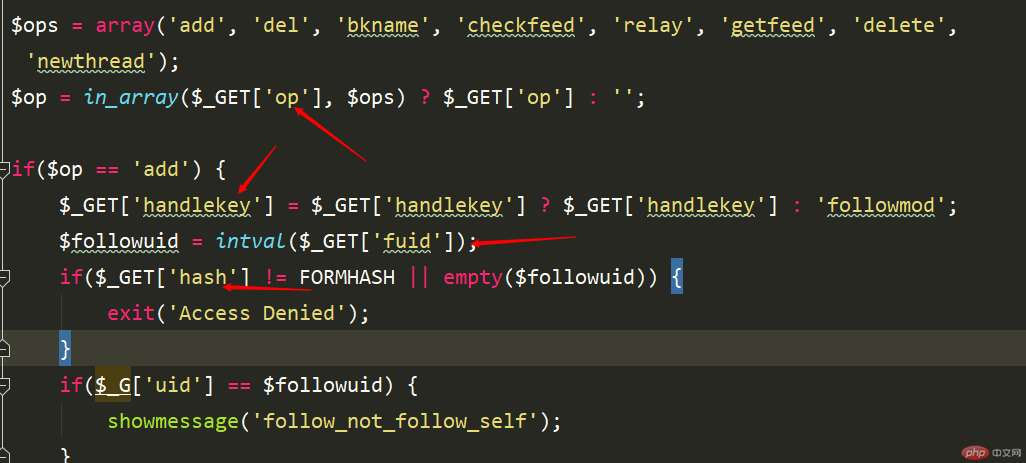```          <a href="home.php?mod=spacecp&ac=follow&op=add&hash={FORMHASH}&fuid={\$space[uid]}&mobile=2
" class="dialog fz12 follow">关注ta
</a>```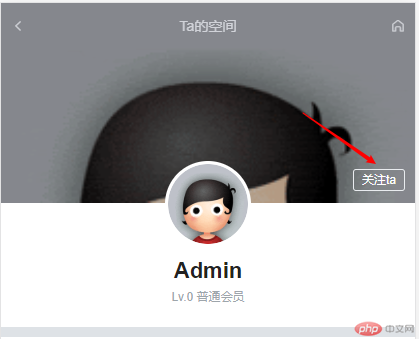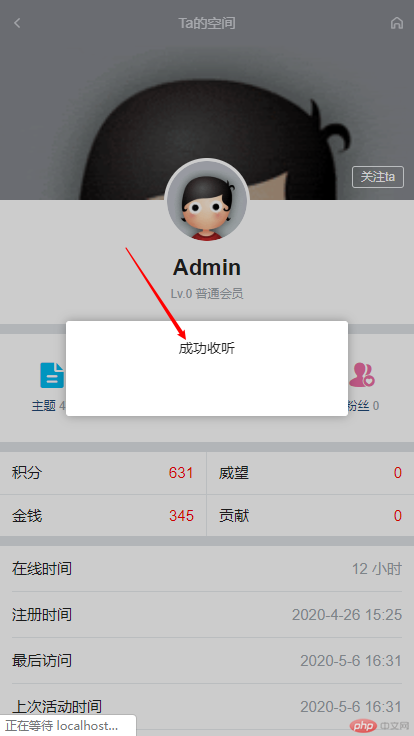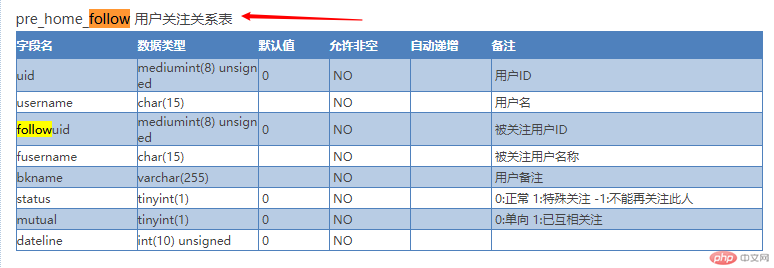## 处理是否显示关注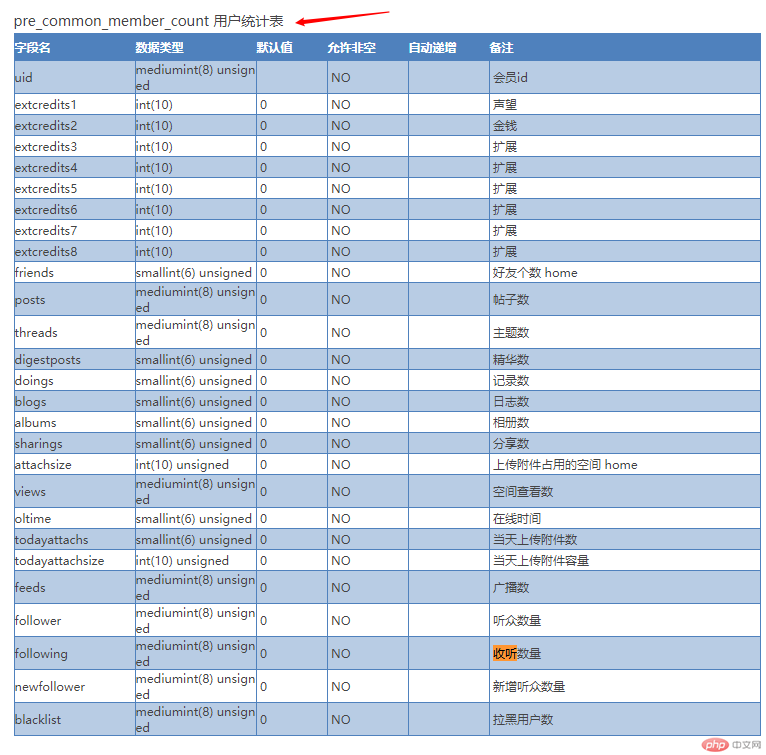```# 判断是否关注
\$follow_data =  DB::fetch_all("select * from pre_home_follow where uid = '\$_G[uid]' and followuid = '\$space[uid]' limit 1");
if(!empty(\$follow_data)){
\$space['is_follow'] = \$follow_data['mutual'];
}```

```<div class="porfile_card_follow cl">
<!--{if isset(\$space[is_follow]) && \$space[is_follow] == 0}-->
<a href="home.php?mod=spacecp&ac=follow&op=del&fuid={\$space[uid]}&mobile=2
" class="dialog fz12 follow">已关注
</a>
<!--{else}-->
" class="dialog fz12 follow">关注ta
</a>
<!--{/if}-->
</div>```

## 测试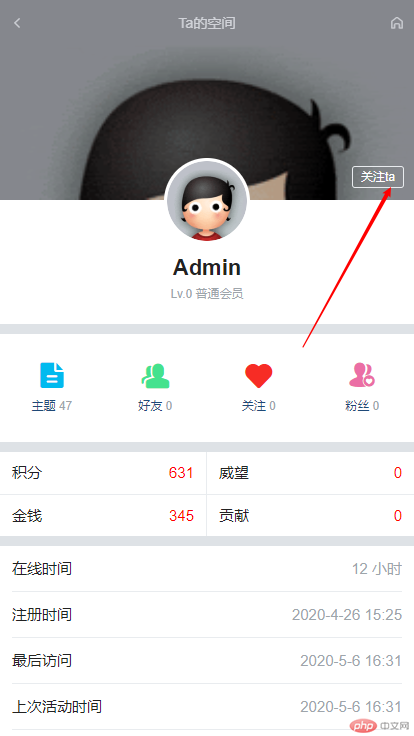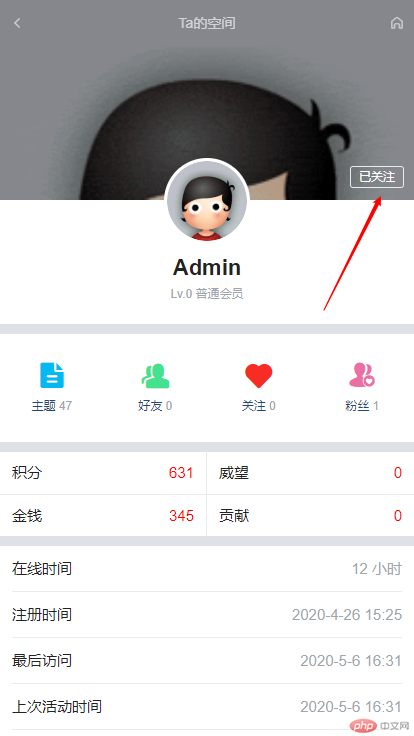推荐文章
• 热门
• 最新